# Risk and Return Expected Return and Variance Expected

• Slides: 19Risk and Return Expected Return and VarianceExpected Return State of Probability of Stock Returns if State Economy State of Economy Occurs Stock A Stock B Boom 50% 0. 70 0. 10 Recessio 50% -0. 20 0. 30 n Which one would you like?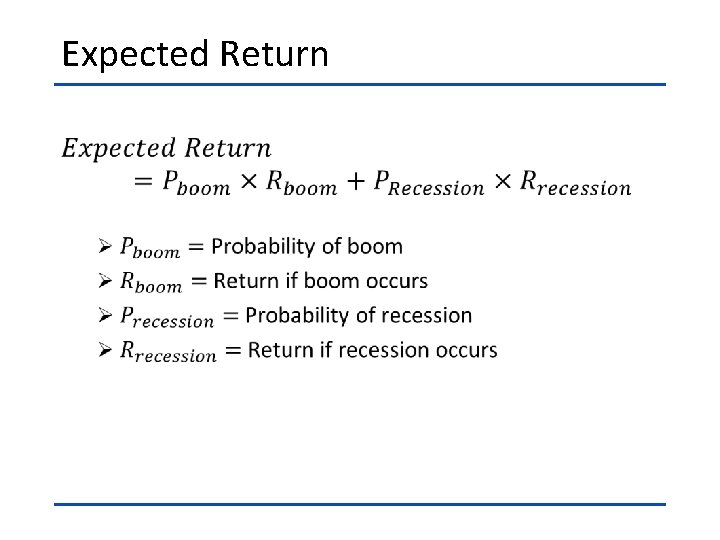Expected Return •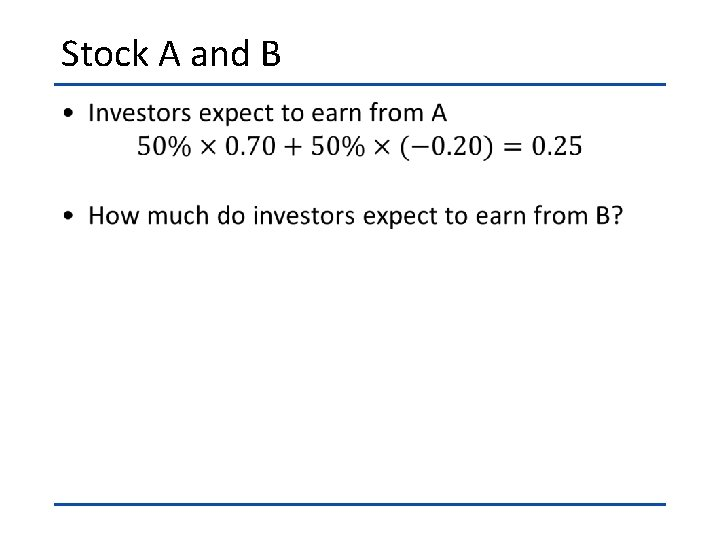Stock A and B •Problem 11 -6 Calculating Expected Return Consider the following information: State of Probability of Econom State of y Economy Recessi 0. 22 on Normal 0. 48 Boom 0. 30 Calculate the expected return. Stock Returns if State Occurs -0. 12 0. 14 0. 33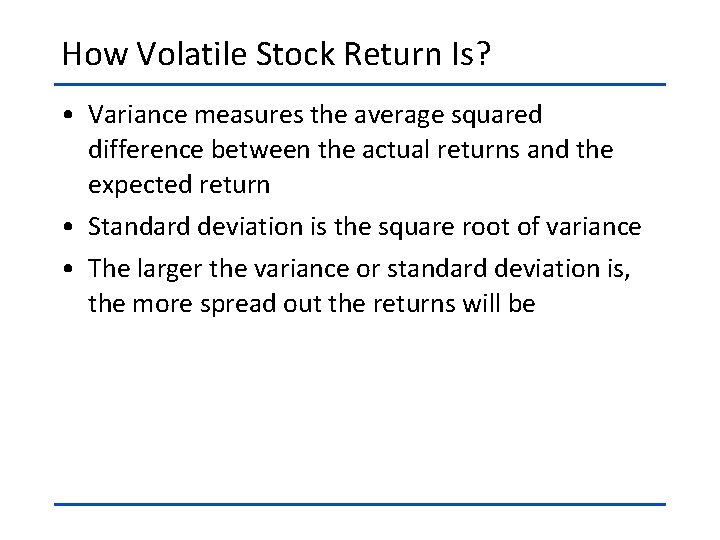How Volatile Stock Return Is? • Variance measures the average squared difference between the actual returns and the expected return • Standard deviation is the square root of variance • The larger the variance or standard deviation is, the more spread out the returns will beVariance and Standard Deviation •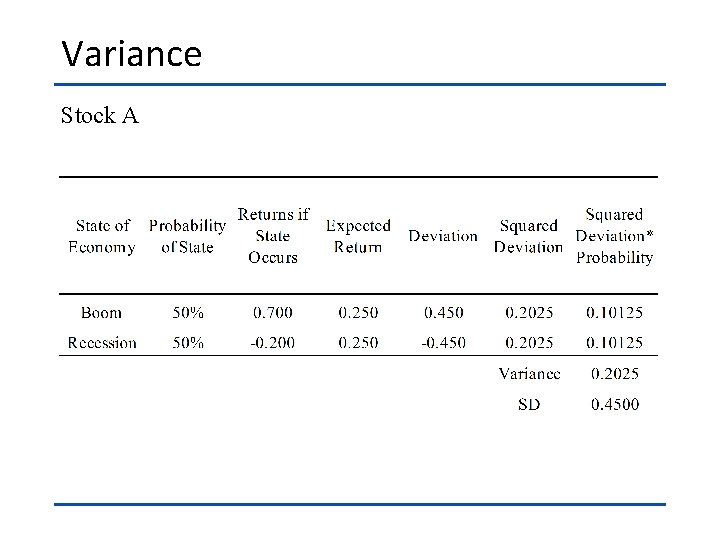Variance Stock A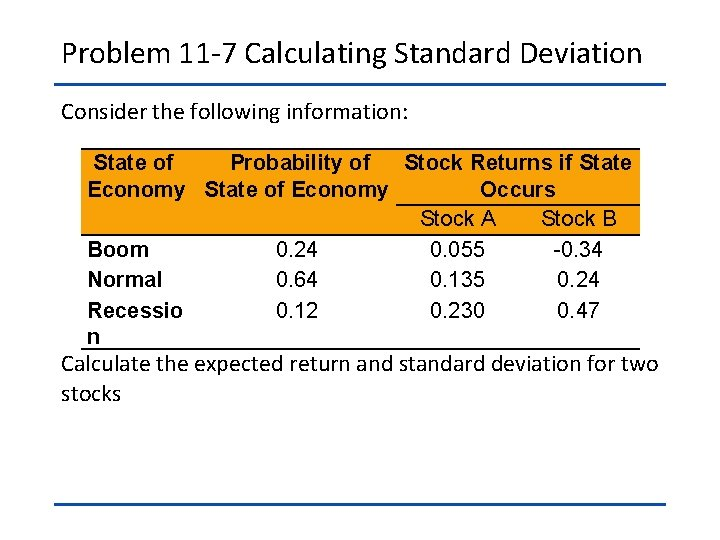Problem 11 -7 Calculating Standard Deviation Consider the following information: State of Probability of Stock Returns if State Economy State of Economy Occurs Stock A Stock B Boom 0. 24 0. 055 -0. 34 Normal 0. 64 0. 135 0. 24 Recessio 0. 12 0. 230 0. 47 n Calculate the expected return and standard deviation for two stocks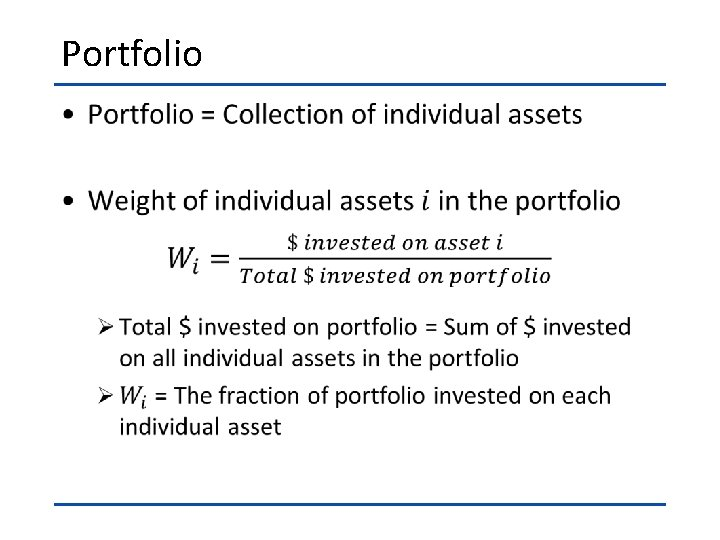Portfolio •If We Invest on A and B… •Problem 11 -1 Determining Portfolio Weights What are the portfolio weights for a portfolio that has 150 shares of Stock A that sell for \$87 per share and 125 shares of Stock B that sell for \$94 per share?Portfolio Expected Return •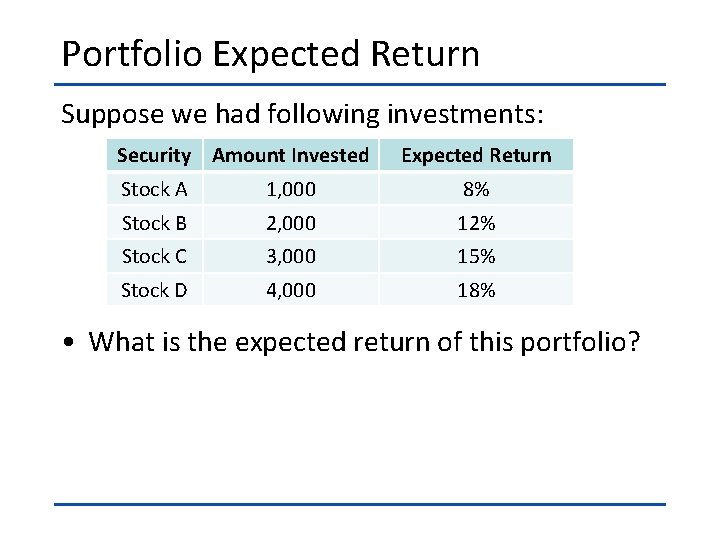Portfolio Expected Return Suppose we had following investments: Security Amount Invested Expected Return Stock A 1, 000 8% Stock B 2, 000 12% Stock C 3, 000 15% Stock D 4, 000 18% • What is the expected return of this portfolio?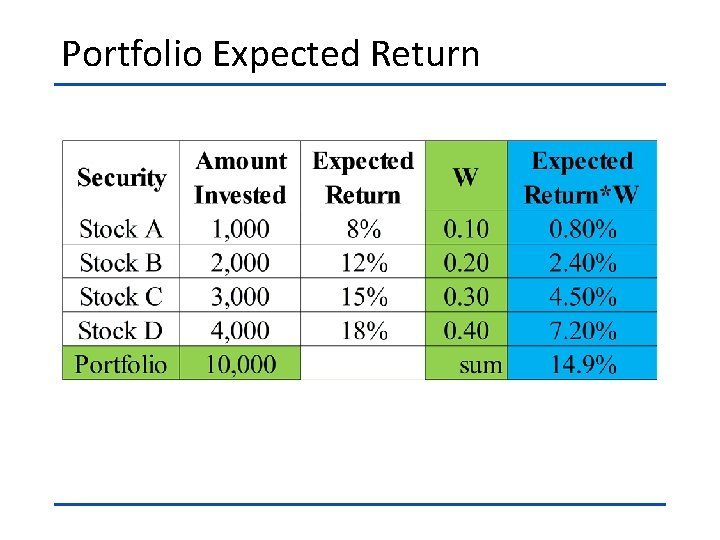Portfolio Expected Return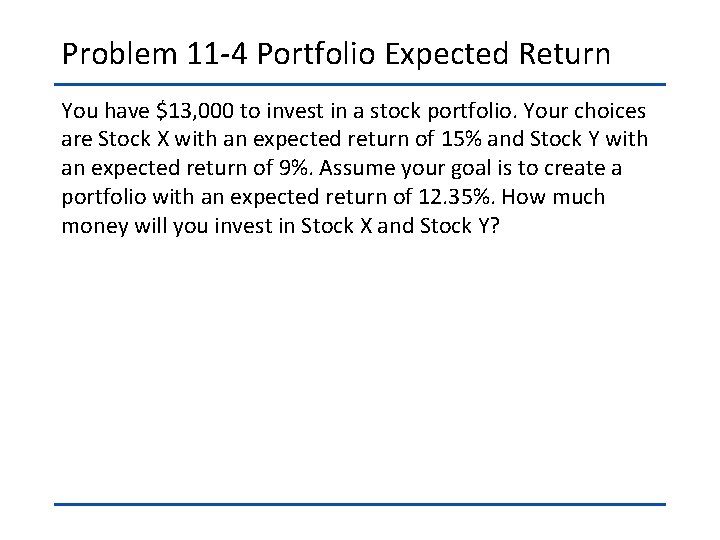Problem 11 -4 Portfolio Expected Return You have \$13, 000 to invest in a stock portfolio. Your choices are Stock X with an expected return of 15% and Stock Y with an expected return of 9%. Assume your goal is to create a portfolio with an expected return of 12. 35%. How much money will you invest in Stock X and Stock Y?Portfolio Variance and Standard Deviation If we invest \$200 on stock A and invest \$800 on B, what is the portfolio variance? State of Probability of Stock Returns if State Economy State of Economy Occurs Stock A Stock B Boom 50% 0. 70 0. 10 Recessio 50% -0. 20 0. 30 nPortfolio Variance and Standard Deviation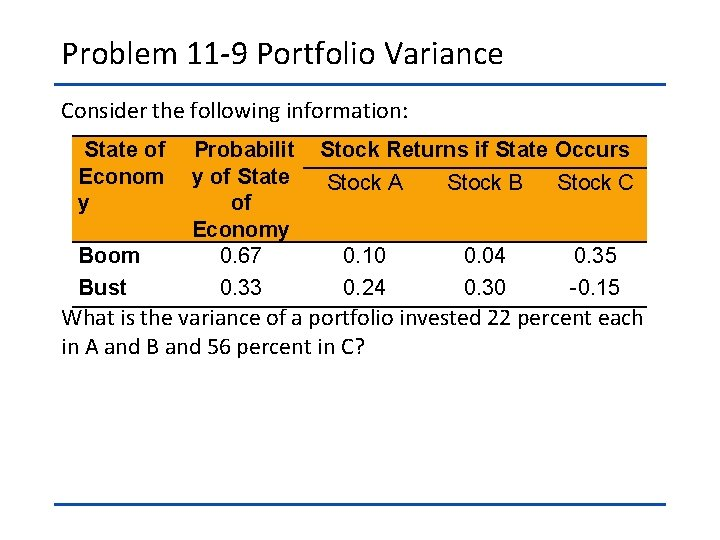Problem 11 -9 Portfolio Variance Consider the following information: State of Probabilit Econom y of State y of Economy Boom 0. 67 Bust 0. 33 Stock Returns if State Occurs Stock A Stock B Stock C 0. 10 0. 24 0. 04 0. 30 0. 35 -0. 15 What is the variance of a portfolio invested 22 percent each in A and B and 56 percent in C?